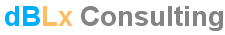Acoustics, Noise Control, Audio Systems and Lighting

General Concepts

Frequency, Octave, and Wavelength
Frequency (f) is the number of cycles that the periodic signal completes in one second. The unit of the frequency is Hz (Hertz). The pure tone or the sine wave has a single frequency. Sound and noise usually are not pure tones.

According to Fourier transform (the complex signal can be synthesized from sine signals/pure tones of different frequencies; different amplitudes; and different time delays/phases) the sound signal represents pure tones with defined amplitudes (or intensities). Tone generator and frequency spectrum of "sound" voice can be found at the Examples page.

The upper and the lower limits of the audible frequency range (generally considered 16 Hz to 20,000 Hz) depend on many factors such as the setup of the measurements and the age of the listeners. The human hearing system (the ears and the related perception system in the brain) is more sensitive to frequencies in the range of 1000 Hz-4000 Hz as shown in the drawing of the next paragraph.

The human hearing system is unable to distinguish between two separate sounds with frequencies too close to each other. In other words, a slight change in the frequency of the tone will not be audible unless the frequency change is greater than a defined value. The higher the frequencies, the broader the frequency change of the tone will be without audible differences. Therefore, the audible frequencies are divided into 220 ranges, the higher the frequency, the wider the range. A unit was defined to describe the concept of these frequency ranges. This unit is called octave which is the interval between two frequencies having a ratio of 2:1. When a greater frequency resolution is needed for some studies, a unit with less value such as 1/3 octave can be used.

The following drawing illustrates some sounds in the frequency domain: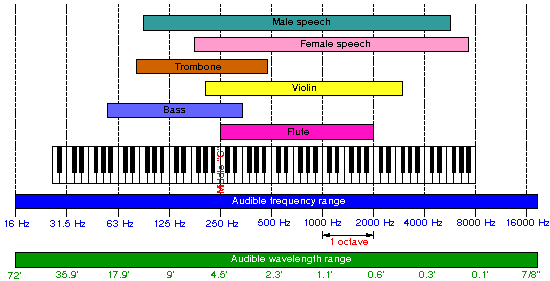Pure tone wave has a frequency, therefore it must has wavelength which is the distance the sound travels in one cycle time. The higher the frequency is, the shorter the wavelength.

Sound Levels and Decibel
The definitions of sound levels and decibel are related to the amplitude of the signal. The absolute value of the sound intensity (I) can be described in Watts per square meter or W/m². As discussed above for frequency, the change in the sound intensity (or sound pressure level, SPL) must be more than a certain value in order to be heard by the human hearing system.

The full range of the audible sound intensity values can be divided into 100 ranges, the higher the sound intensity (louder the sound), the wider the intensity range. Taking into account this human perception, the unit Bel is used. Bel is the logarithm of the ratio of two power values. If, for example, intensity I=0.1 W/m² change to 1 W/m², the resulting sound level (note that the word "level" is added for logarithmic values) will be 1 Bel considering 0.1 W/m² as the reference value (to take the log). Hence the Bel unit is considerably large, one tenth of it is used (the same concept as discussed above for more frequency resolution), and the resulting unit is called decibel or (dB).

In sound application the dB reference value is 20 μPa for sound pressure or 10E-12 W/m² for sound intensity. These values represent 0 dB sound level and the human threshold of hearing (the lowest level that can be perceived). The threshold of pain or feeling (the level which causes pain in the ears) is about 120 dB as shown in the following drawing: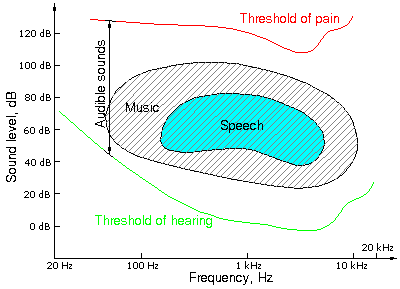Note that the decibel values of sounds cannot be added to obtain the value of the resulting sound. Instead, the combining levels are turned into intensities, added together and then turned back into decibel values.

Sound level 1 dB + Sound level 2 dB      dB

Sound Absorption Coefficient
Sound absorption coefficient describes the efficiency of the material or the surface to absorb the sound. The ratio of the absorbed sound energy to the incident energy is the sound absorption coefficient.

For architectural purposes, sound absorbing materials and constructions can be divided into four types of materials depending on the way the absorption is mainly performed:
1-Turning the sound energy into heat such as fiberglass and carpet.
2-Vibrating with a specific frequency when the sound hits the surface such as lightweight panels and 5/8" gypsum board. (These materials absorb the sound effectively on a narrow band of frequencies)
3-Turning the sound energy into heat in the neck of the cavities (Helmholtz resonator) such as sound blocks (this construction has a good absorption on low frequencies).
4-Allowing the sound to go through such as some types of grid systems and lay-in ceiling with sound leakage above it.

The most common way to measure sound absorption coefficient is to lay a piece of the material in a reverberant room (a room which has very long Reverberation Time) then measure the RT so the coefficient can be derived from Sabin equation (the original version of RT calculation). There is a standard that details this procedure.

The value of the absorption coefficient for the same material varies with the type of the mounting in the test room. Mounting types that are frequently given in manufacturer's data sheets for the acoustical panels are illustrated in the following drawing: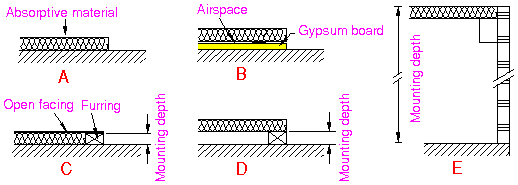Noise reduction coefficient NRC is the arithmetic average of the sound absorption coefficients at 250, 500, 1000, and 2000 Hz, then this average is rounded to the multiples of 0.05.

Diffraction
Diffraction is the change in the direction of the propagation of sound waves passing the edge of the obstacle as illustrated in the following figure: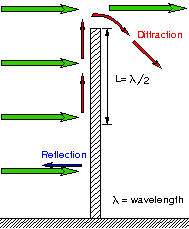Diffraction phenomenon depends significantly on the ratio of the wavelength of the sound to the size of the obstacle. The longer the wavelength is, the stronger the sound diffraction. Diffraction effect happens to sounds transmitted through openings as well.